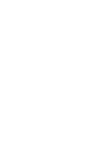# ICMS 2016

The 5th International Congress on Mathematical Software
 Invited Speakers Prof. Wolfram Decker (University of Kaiserslautern) Current Challenges in the Development of Open Source Computer Algebra Software Abstract: Computer algebra is facing new challenges as more and more of the abstract concepts of pure mathematics are made constructive, with interdisciplinary methods playing a significant role. On the mathematical side, while we wish to provide cutting-edge techniques for applications in various areas, the implementation of an advanced and more abstract computational machinery often depends on a long chain of more specialized algorithms and efficient data structures at various levels. On the software development side, for cross-border approaches to solving mathematical problems, the efficient interaction of systems specializing in different areas is indispensable. In this talk, I will report on ongoing collaboration between groups of developers of several well-established open source computer algebra system specializing in commutative algebra and algebraic geometry, group and representation theory, convex and polyhedral geometry, and number theory. The ultimate goal of this collaboration is to integrate the systems, together with other packages and libraries, into a next generation computer algebra system surpassing the combined mathematical capabilities of the underlying systems. Slides of the talk Prof. Jack Dongarra (University of Tennessee) With Extreme Scale Computing the Rules Have Changed Abstract: In this talk we will look at the current state of high performance computing and look at the next stage of extreme computing. With extreme computing there will be fundamental changes in the character of floating point arithmetic and data movement. In this talk we will look at how extreme scale computing has caused algorithm and software developers to changed their way of thinking on how to implement and program certain applications. Slides of the talk Prof. Vladimir Voevodsky (IAS Princeton) UniMath - a library of mathematics formalized in the univalent style. Abstract: The univalent style of formalization of mathematics in the type theories such as the ones used in Coq, Agda or Lean is based on the discovery in 2009 of a new class of models of such type theories. These “univalent models” led to the new intuition that resulted in the introduction into the type theory of the concept of h-level (homotopy level). This most important concept implies in particular that to obtain good intuitive behavior one should define propositions as types of h-level 1 and sets as types of h-level 2. Instead of syntactic Prop one then *defines* a type hProp(U) - the type of types of h-level 1 in the universe U and the type hSet(U) - the type of types of h-level 2 in U. With types of h-level 1 and 2 one can efficiently formalize all of the set-theoretic mathematics. With types of h-level 3 one can efficiently formalize mathematics at the level of categories etc. Univalent style allows to directly formalize constructive mathematics and to formalize classical mathematics by adding the excluded middle axiom for types of h-level 1 and the axiom of choice for types of h-level 2. UniMath is a growing library of constructive mathematics formalized in the univalent style using a small subset of Coq language. Slides of the talk Prof. Stephen M. Watt (University of Waterloo) Toward an International Mathematical Knowledge Base The notion of a comprehensive digital mathematics library has been a dream for some decades. More than in many other areas, results in mathematics have lasting value--once proven, always true. It is not uncommon for a research article to have primary references to work decades earlier. Another quality of mathematics is its precision; there is a clarity to mathematical definitions and results. This makes mathematics an ideal subject for mechanized treatment of knowledge. This talk shall outline the challenges and opportunities in transforming the complete mathematical literature into a knowledge base to be used by mathematicians and software systems alike.